Next: 9.3.4 Handling Multiple Devices Up: 9.3 Inverse Modeling of Previous: 9.3.2 Modeling the Doping

## 9.3.3 Deriving a Metric for the Match

The network-model described by Example 9.4 and depicted in Figure 9.6 quantifies the match for the transfer (ID/VG), and output curves (ID/VD) for the NMOS device produced by the simulation-flow-model designed before. It uses MINIMOS-NT for this purpose, which is encapsulated by a simulation-flow-model ("device.mod"). MINIMOS-NT offers a nice feature to read the sequence of operating points to be simulated, which constitute a desired I/V-curve, from a preexisting I/V-curve. Since this model has to compare these curves to the measured I/V-curves, we use the settings of the measurements to control the MINIMOS-NT device simulator. Thus, we derive simulated I/V-curves which are structurally identical to their measured equivalent.

The actual comparison is done by the curve-calculator-model ("curve-calc.mod") described by Example 9.3. It defines the measure of match as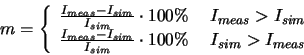which delivers values between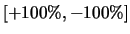. The output of the curve-calculator-model is a curve which contains this measure of match for each operating point of the measured curve.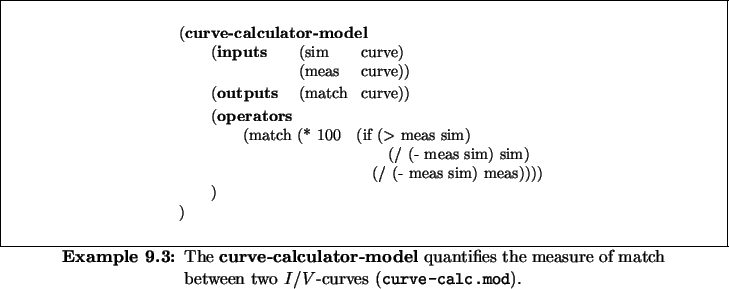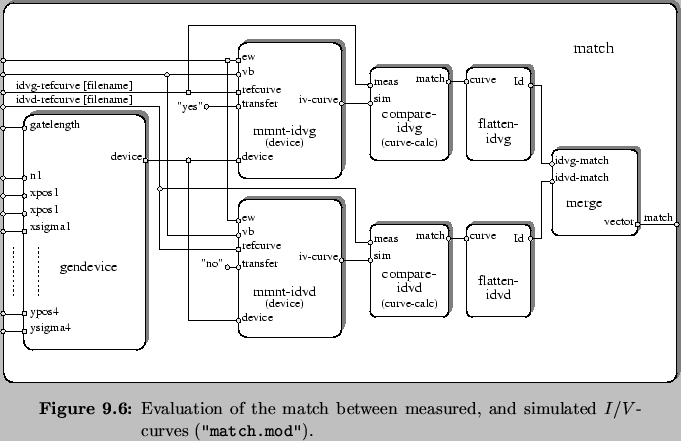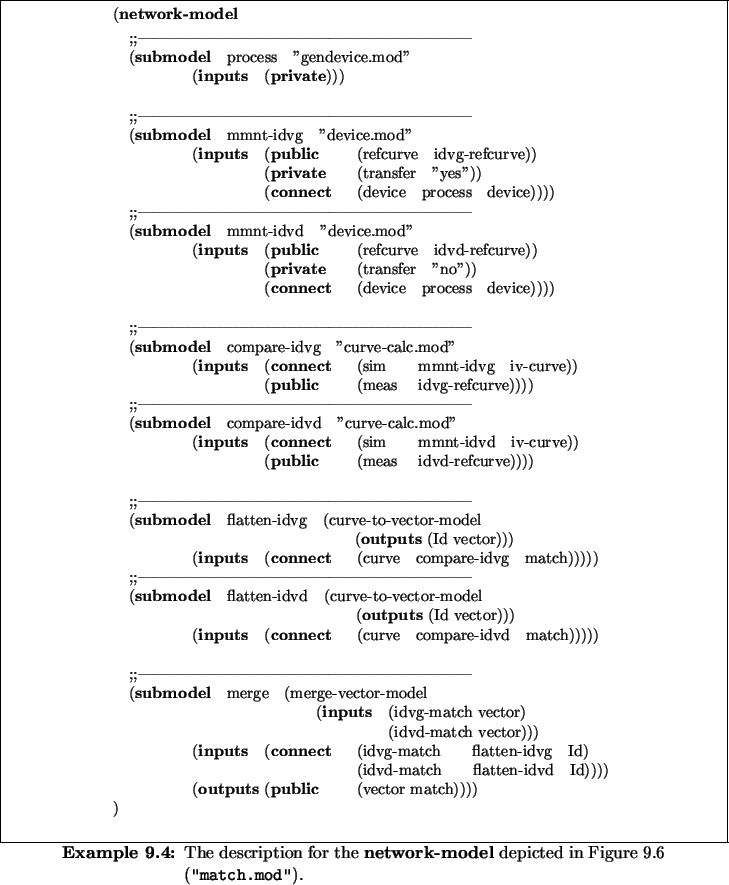As next step we convert this structured representation of the match into the flat representation of a vector of floating point numbers. We do this by means of the curve-to-vector-models labeled flatten-idvg, and flatten-idvd. These models convert the curve column known as Id into a vector. Finally, we concatenate these two vectors into a single one by means of a merge-vector-model, which is labeled merge and thus obtain the result of the network-model at the output port named match.Next: 9.3.4 Handling Multiple Devices Up: 9.3 Inverse Modeling of Previous: 9.3.2 Modeling the Doping
Rudi Strasser
1999-05-27